Addition - Definition with Examples

The Complete K-5 Math Learning Program Built for Your Child

• 30 Million Kids

Loved by kids and parent worldwide

• 50,000 Schools

Trusted by teachers across schools

• Comprehensive Curriculum

Aligned to Common Core

The addition is taking two or more numbers and adding them together, that is, it is the total sum of 2 or more numbers.

Example:

How many apples are there in all?There are 7 apples in one basket and 4 apples in the other. So, we add 7 and 4 to find the total number of apples.

To add 7 and 4, we can count forward 4 steps from 7

The symbol used to indicate Addition is + (plus symbol).

So, 7 and 4 can be written as 7 + 4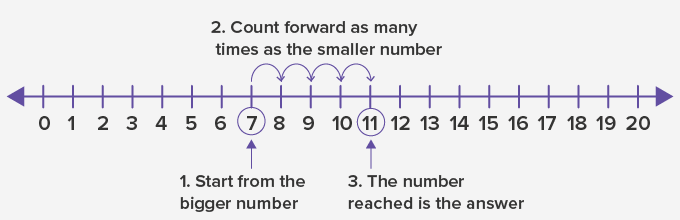An addition sentence is a mathematical expression that shows two or more values added together and their sum.

We can write the mathematical expression for 7 plus 4 equals 11 as: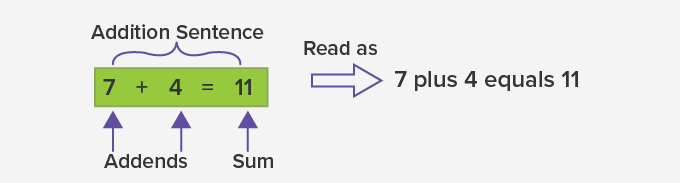The numbers that are added are called addends and the answer to addition is called the sum. In an addition sentence, the addends are added to get the sum.

Addition of small numbers can be done using your fingers too.

Examples: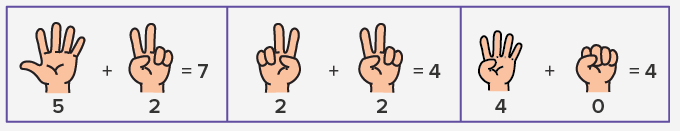Number charts are another way to add numbers.

Example: Add 57 and 16 using a hundred grid.

 Step 1: Mark the bigger number. (Here, mark 57) Step 2: If the number to be added is more than 10, break it in tens and ones. (Here, 16 = 10 + 6) Step 3: Jump as many 10s as in the second number. (Here, 57 + 10 = 67)  Step 4: Move forward as many ones as in the second number. (Here, 67 + 6 = 73) The number reached is the answer.   So, 57 + 16 = 73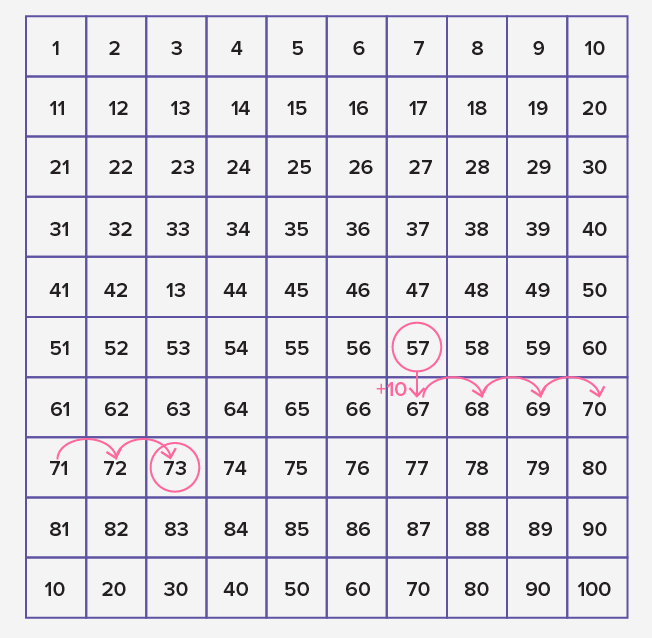Numbers can also be added vertically.

Let’s add 57 and 16 vertically.

 Step 1: Write the numbers one below the other as per the places of the digits. Step 2: Start adding from the ones digit. Write the sum under the ones digit. If the sum of the ones digit is greater than 9, write the ones digit of the sum under the ones and carry forward its tens digit to the tens column. Step 3: Add the tens digits. (If there was a carry forward digit, add it along)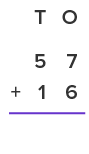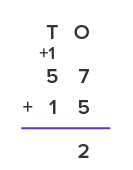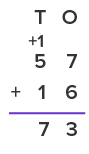The numbers with more than 2 digits can also be added vertically. We always start adding from the ones digit and move towards the digits at the highest place.

Example 1: Marie bought a designer handbag for \$231 and a belt for \$199. How much did she spend on both?

To find the total cost, we need to add 231 and 199

We can add the numbers vertically as: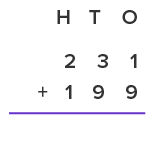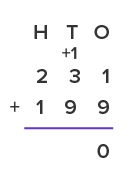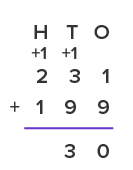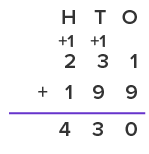So, Marie spent \$430

Example 2: There are 2415 blue seats and 2770 red seats in a stadium. How many blue and red seats are there altogether?

Total number of seats = 2415 + 2770

= 5185 seats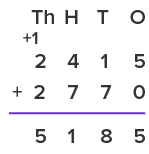Fun Facts Adding 0 to any number gives the sum as the number itself. Adding 1 to a number repeatedly is the same as counting.

Won Numerous Awards & Honors